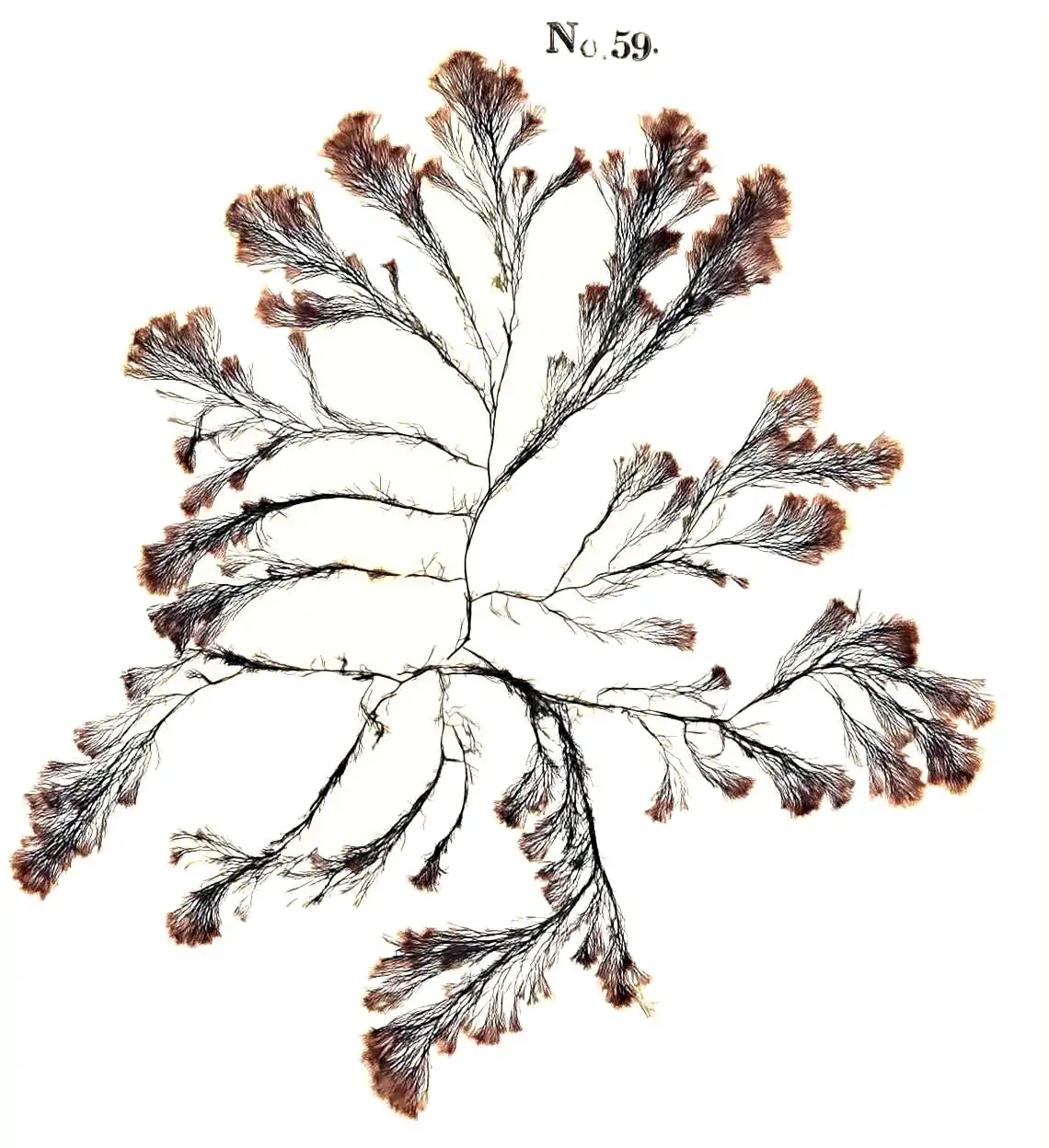## a.k.a. cluster distributions, Galton-Watson models$$\newcommand{\rv}{\mathsf{#1}}$$

Models for, loosely, the total population size arising from all generations the offspring of some progenitor.

Let us suppose that each individual $$i$$ who catches a certain strain of influenza will go on to infect a further $$\rv{n}_i\sim F$$ others. Assume the population is infinite, that no one catches influenza twice and that the number of transmission of the disease is distributed the same for everyone who catches it. How many people, will ultimately catch the influenza, starting from one infected person?

The Galton-Watson version of this model considers this byu generation; We write $$\rv{x}(k)=\sum_{i \in k\text{th generation}} \rv{n}_i$$ for the number of people infected in the $$k$$th generation. Writing $$F^{*k}$$ for the $$k$$-fold convolution of $$F$$, we have $\rv{x}(k) \sim F^{\ast \rv{x}(k-1)}$ The sum over all these $\sum_k \rv{x}(k)$ is the cascade size.

A type of count model for a Markov stochastic pure-birth branching process.

I say it is a count model, but it turns out there are continuous-state generalisations. See, e.g. .

The distribution of subcritical processes are sometimes tedious to calculate, although we can get a nice form for the generating function of a geometric offspring distribution cascade process.

Set $$\frac{1}{\lambda+1}=p$$ and $$q=1-p$$. We write $$G^{n}\equiv G\cdot G\cdot \dots \cdot G\cdot G$$ for the $$n$$-fold composition of $$G$$. Then the (non-critical) geometric offspring distribution branching process obeys the identity

$1-G^n(s;\lambda) = \frac{\lambda^n(\lambda-1)(1-s)}{\lambda(\lambda^n-1)(1-s)+\lambda-1}$

This can get us a formula for the first two factorial moments, and hence the vanilla moments and thus mean and variance etc

More generally the machinery of Lagrangian distributions is all we need to analyse these.

Maybe I should use to get the moments? Dominic Yeo explains beautifully as always.

🏗 🏗 🏗

## Lagrangian distributions

A clade of count distributions, which I would call “cascade size distribution”. For now, let’s get to the interesting new ones contained in this definition. They are unified to my mind by modelling cascade size of cluster processes. Specifically, if I have a given initial population and a given offspring distribution for some population of… things… a Lagrangian distribution gives me a model for the size of the total population. There are other interpretations of course (queueing is very popular), but this one is extremely useful for me. See for a deep dive on this. They introduce various exponential_families via the pgf, which is powerful and general, although it does obscure a lot of simplicity and basic workaday mathematics where the forms of the mass functions do in fact turn out to be easy.

Terminology: the total cascade size of a subcritical branching process has a “delta Lagrangian” or “general Lagrangian” distribution, depending on whether the cluster has, respectively, a deterministic or random starting population. We define the offspring distribution of such a branching process as $$G\sim G_Y(\eta, \alpha)$$. Usually we also assume $$EG:=\eta< 1$$, because otherwise the cascade size is infinite.

### Borel-Tanner distribution

A delta Lagrangian distribution, the Borel distribution is the distribution of a cascade size starting from a population size of $$k=1$$. We can generalize it to $$k>$$, in which case it is the Borel-Tanner distribution.

Spelled
$$\operatorname{Borel-Tanner}(k,\eta)$$
Pmf
((X=x;k,)={}{}}
Mean
$$\frac{k}{1-\eta}$$
Variance
$$\frac{k\eta}{(1-\eta)^3}$$

Note to self: Wikipedia mentions an intriguing-sounding correspondence with random walks, which I should follow up Dwass (1969).

The only R implementation I could find for this is in VGAM, although it is not so complicated.

### Poisson-Poisson Lagrangian

See (P. C. Consul and Famoye 2006 Ch 9.3). Also known as the Generalised Poisson, although there are many things called that.

Spelled
$$\operatorname{GPD}(\mu,\eta)$$
Pmf
$$\mathbb{P}(X=x;\mu,\eta)=\frac{\mu(\mu+ \eta x)^{x-1}}{x!e^{\mu+x\eta}}$$
Mean
$$\frac{\mu}{1-\eta}$$
Variance
$$\frac{\mu}{(1-\eta)^3}$$

Returning to the cascade interpretation: Suppose we have

• an initial population is distributed $$\operatorname{Poisson}(\mu$$)
• and everyone in the population has a number of offspring distributed $$\operatorname{Poisson}(\eta$$).

Then the total population is distributed as $$\operatorname{GPD}(\mu, \eta)$$.

Notice that this can produce long tails, in the sense that it can have a large variance with finite mean, but not heavy tails, in the sense of the variance becoming infinite while retaining a finite mean; both variance and expectation go to infinity together.

Here, I implemented the GPD for you in python. There are versions for R, presumably. A quick search turned up RMKDiscrete and LaplacesDemon.

### General Lagrangian distribution

A larger family of Lagrangian distributions (the largest?) family is summarised in , in an unintuitive (for me) way.

One parameter: a differentiable (infinitely differentiable?) function, not necessarily a pgf, $$g: [0,1]\rightarrow \mathbb{R}$$ such that $$g(0)\neq 0\text{ and } g(1)=1$$. Now we define a pgf $$\psi(s)$$ implicitly as the smallest root of the Lagrange transformation $$z=sg(z)$$. The paradigmatic example of such a function is $$g:z\mapsto 1−p+pz$$; let’s check how this fella out.

🏗

Spelled
?
Pmf
?
Mean
?
Variance
?

### No comments yet. Why not leave one?

GitHub-flavored Markdown & a sane subset of HTML is supported.